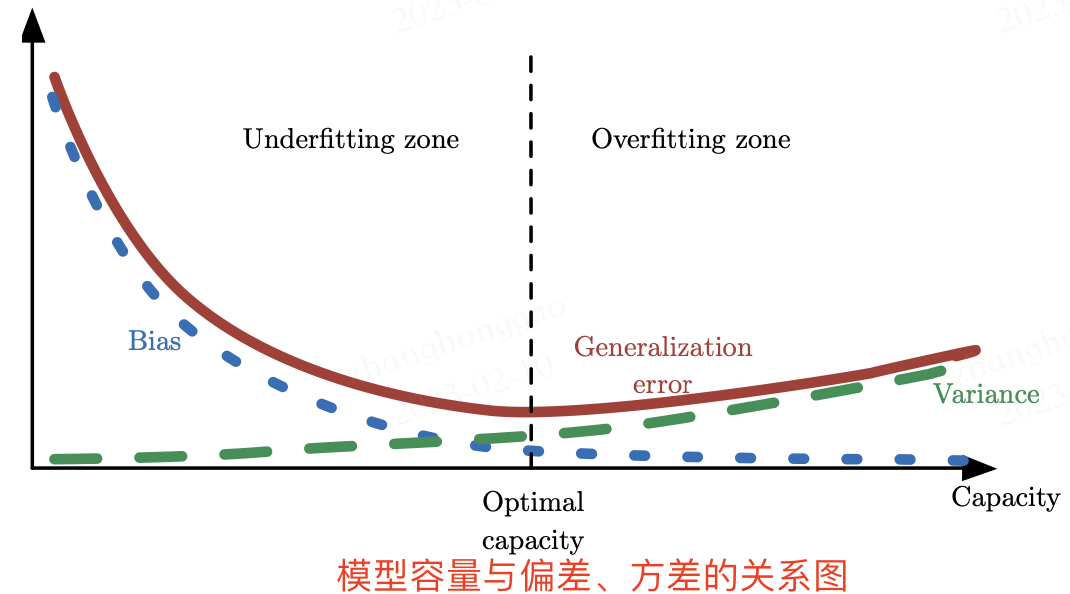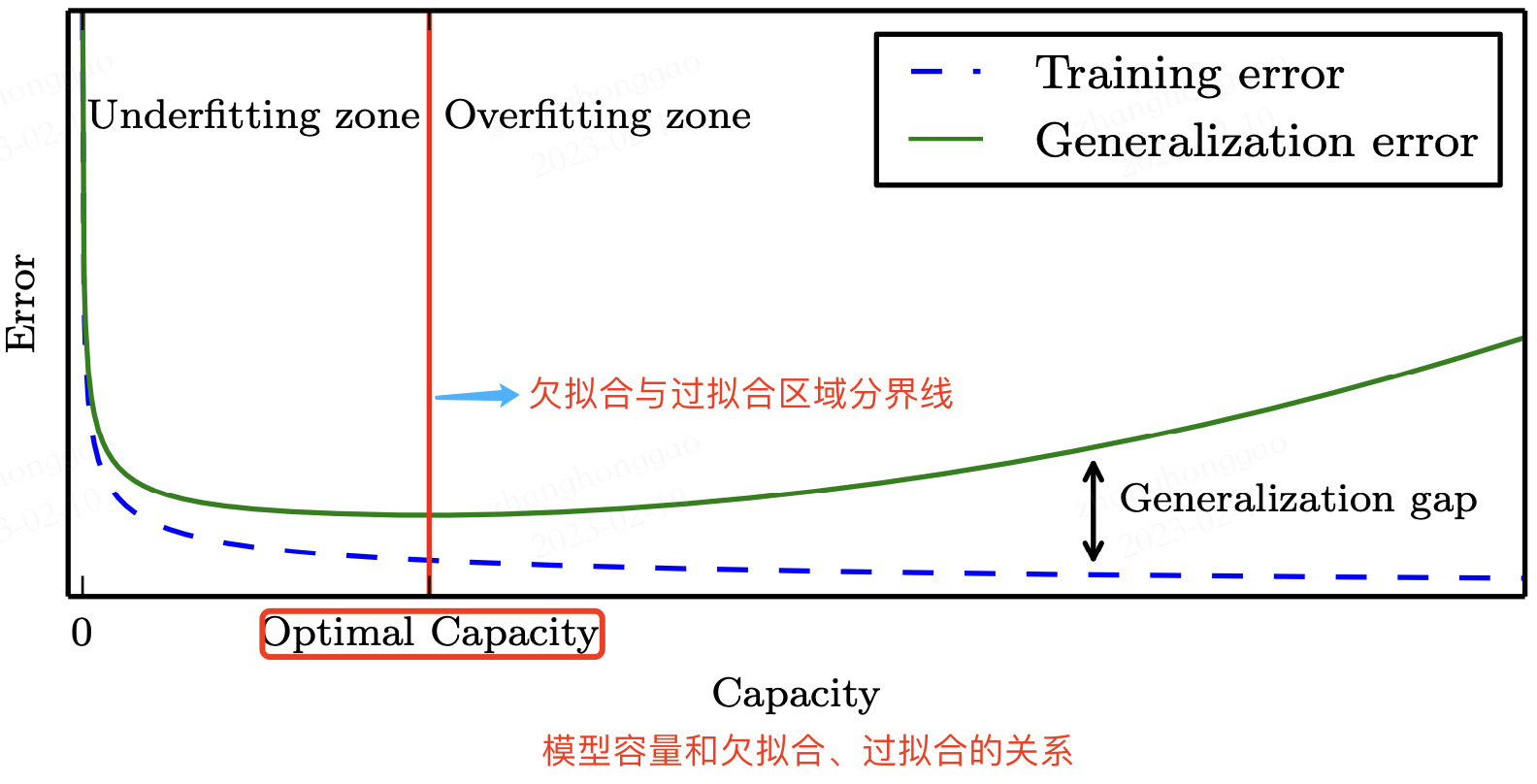# 机器学习基本概念总结

## 一，余弦相似度与欧氏距离

### 1.1，余弦相似度

• 当两个向量夹角越大，距离越远，最大距离就是两个向量夹角 180°；
• 夹角越小，距离越近，最小距离就是两个向量夹角 0°，完全重合。
• 夹角越小相似度越高，但由于有可能一个文章的特征向量词特别多导致整个向量维度很高，使得计算的代价太大不适合大数据量的计算。

$$cos(\theta) = \frac {ab}{||a|| \times ||b||} = \frac {x_{1}x_{2}+y_1y_2}{\sqrt{x^2_1+y^2_1}\sqrt{x^2_2+y^2_2}}$$

（分子就是两个向量的内积，分母是两个向量的模长乘积）

### 1.2，欧式距离

$$d(x,y) = \sqrt {((x_{1}-y_{1})^{2} + (x_{2}-y_{2})^{2} + ... + (x_{n}-y_{n})^{2})}$$

### 1.3，余弦相似度和欧氏距离的区别

• 欧式距离和余弦相似度都能度量 2 个向量之间的相似度
• 放到向量空间中看，欧式距离衡量两点之间的直线距离，而余弦相似度计算的是两个向量之间的夹角
• 没有归一化时，欧式距离的范围是 [0, +∞]，而余弦相似度的范围是 [-1, 1]；余弦距离是计算相似程度，而欧氏距离计算的是相同程度（对应值的相同程度）
• 归一化的情况下，可以将空间想象成一个超球面（三维），欧氏距离就是球面上两点的直线距离，而向量余弦值等价于两点的球面距离，本质是一样。

## 2.1，概念定义

$$Error = Bias + Varience$$

Varience(Error due to Variance), Bias(Error due to Bias)

• Error: 反映的是整个模型的准确度。
• Bias：偏差引起的误差被视为我们模型的预期（或平均）预测与我们试图预测的正确值之间的差异，即模型的准确性。当然，您只有一个模型，因此谈论预期或平均预测值可能看起来有点奇怪。但是，想象一下您可以多次重复整个模型构建过程：每次您收集新数据并运行新分析时都会创建一个新模型。由于基础数据集中的随机性，生成的模型将具有一系列预测。偏差通常衡量这些模型的预测与正确值之间的差距。
• Varience：方差引起的误差被视为给定数据点的模型预测的可变性，即**即模型的稳定性。同样，假设您可以多次重复整个模型构建过程。方差是给定点的预测在模型的不同实现之间的变化程度。

### 2.2，图形定义

BiasVarience 的图形定义：

Low Bias 表现出来就是点都打在靶心中间，但这样容易过拟合 (overfitting)，过拟合对应下图是 High Variance，表现就是点很分散，没有集中在一起，手不稳啊（对应就是模型预测结果变化性太大）。 Low Variance 对应就是点都打的很集中，但不一定是靶心附近，手很稳，但是瞄的不一定准。### 2.4，导致偏差和方差的原因

• 偏差通常是由于我们对学习算法做了错误的假设，或者模型的复杂度不够；
• 比如真实模型是一个二次函数，而我们假设模型为一次函数，这就会导致偏差的增大（欠拟合）；
• 由偏差引起的误差通常在训练误差上就能体现，或者说训练误差主要是由偏差造成的
• 方差通常是由于模型的复杂度相对于训练集过高导致的；
• 比如真实模型是一个简单的二次函数，而我们假设模型是一个高次函数，这就会导致方差的增大（过拟合）；
• 由方差引起的误差通常体现在测试误差相对训练误差的增量上。

### 2.5，深度学习中的偏差与方差

• 神经网络的拟合能力非常强，因此它的训练误差（偏差）通常较小；
• 但是过强的拟合能力会导致较大的方差，使模型的测试误差（泛化误差）增大；
• 深度学习的核心工作之一就是研究如何降低模型的泛化误差，这类方法统称为正则化方法

## 三，模型容量、过拟合和欠拟合

• 模型容量是指模型拟合各种函数的能力，决定了模型是欠拟合还是过拟合。
• 欠拟合就是指模型的训练误差过大，即偏差（bias）过大，表现为模型不够”准“，优化算法目的在于解决欠拟合问题。
• 过拟合就是指训练误差和测试误差间距过大，即方差（variance）过大，表现为模型不够”稳“，正则化目的在于解决过拟合问题。
• 机器学习模型的目的是解决欠拟合和过拟合的问题，这也是机器学习算法的两个挑战。## 四，样本方差与总体方差

### 4.1，方差定义

1，均方误差（MSE，mean squared error）与均方根误差(RMSE)

$$\frac{1}{n} \sum_{i=1}^{n}[f(x_i)-y_i]^2$$

2，总体方差

$$\sigma ^2 = \frac{\sum_{i=1}^{N}(X_{i}-\mu)^2}{N}$$

3，样本方差

$$\sigma ^2 = \frac{\sum_{i=1}^{n-1}(X_{i}-\overline{x_{i}..x_{n}})^2}{n-1}$$

$\overline{x_{i}..x_{n}}$ 表示样本均值公式分母由总体方差的 N 变为了 n-1，使得样本方差更能反映总体方差。

## 五，先验概率与后验概率

### 5.1，条件概率

$$P(A|B) = \frac {P(A\cap B)} {P(B)}$$

### 5.3，后验概率

• 事情已经发生，要求这件事情发生的原因是由某个因素引起的可能性的大小（由果推因：就是在知道“果”之后，去推测“因”的概率）
• 后验概率和和先验概率的关系可以通过贝叶斯公式求得，公式如下： $$P(B_{i}|A) = \frac {P(B_{i}\cdot P(A|B_{i}}{P(B_{1})\cdot P(A|B_{1}) + P(B_{2})\cdot P(A|B_{2}) }$$

### 5.4，贝叶斯公式

• Bi 常被视为导致试验结果A发生的”原因“；
• P(Bi)(i=1,2,...) 表示各种原因发生的可能性大小，故称先验概率；
• P(Bi|A)(i=1,2...) 则反映当试验产生了结果A之后，再对各种原因概率的新认识，故称后验概率。

### 5.5，后验概率实例

$$P(A|B) = \frac {P(B|A)P(A)}{P(B)} = \frac {0.5\times 0.4} {0.8}$$

## 六，相对熵(KL散度)与交叉熵

### 6.1，信息熵

$$H(P) = \sum_{i} P(i)log_{a} \frac{1}{P(i)} = -\sum_{i}P(i)log_{a} P(i)$$

$P_{i}$ 表示第 $i$ 个事件发生得概率，总的来说信息熵其实从某种意义上反映了信息量存储下来需要多少存储空间。 总结为：根据真实分布，我们能够找到一个最优策略，以最小的代价消除系统的不确定性（比如编码），而这个代价的大小就是信息熵

### 6.2，相对熵/KL散度

KL 散度，有时候也叫 KL 距离，一般被用于计算两个分布之间的不同，记为 $D_{KL}(P||Q) = H(P,Q) - H(P)$，对于同一个离散随机变量 $\textrm{x}$ 有两个单独的概率分布 $P(x)$ 和 $Q(x)$，其 KL 散度为：

$$D_{KL}(P \| Q) = \sum_i P(i)log_{a} \frac{P(i)}{Q(i)} = \sum_i P(i)[logP(x) - log Q(x)]$$

### 6.3，交叉熵 cross-entroy

• 非负。
• KL 散度相同，交叉熵也不具备对称性，即 $H(P,Q)≠H(Q,P)$。
• 交叉熵主要用于描述两个事件之间的相互关系，对同一个分布求交叉熵等于对其求熵

### 6.5，KL 散度与交叉熵的关系

• KL 散度和交叉熵在特定条件下等价
• $D_{KL}(P||Q) = H(P,Q) - H(P)$

## 七，随机梯度下降算法

• 随机梯度下降算法是目前最为广泛应用的一种神经网络优化算法，形式为 $θ=θ − ϵg$，$ϵ$ 是学习率，$g$ 是梯度，$θ$ 是权重。
• 随机梯度下降优化算法不一定能保证在合理的时间内达到一个局部最小值，但它通常能及时地找到代价函数一个很小的值，并且是有用的。

### 八，超参数和验证集

• 普通参数指算法权重 $w$ 的值，是可以通过学习算法本身学习得到。超参数的值不是通过学习算法本身学习出来的，可通过验证集人为选择合适的超参数
• 将训练数据划分为两个不相交的子集，即训练集和验证集，训练集用于学习普通参数，验证集用于估计训练中或训练后的泛化误差，更新超参数（“训练超参数”）。通常，80% 的训练数据用于训练，20% 用于验证。
• 交叉验证方法适合小规模数据集（例如几百上千张图片）训练模型的情况。

## 九，正则化方法

$$\tilde{J}(\theta; X, y) = J(\theta; X, y) + \alpha \Omega(\theta)$$

• L1 范数: 为向量 x 各个元素绝对值之和。
• L2 范数: 为向量 x 各个元素平方和的 1/2 次方，L2 范数又称 Euclidean 范数或 Frobenius 范数
• Lp 范数: 为向量 x 各个元素绝对值 $p$ 次方和的 $1/p$ 次方.

L1 范数可以使权值参数稀疏，方便特征提取。L2 范数可以防止过拟合，提升模型的泛化能力。

「其他文章」